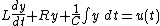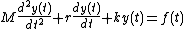# forced oscillation

## Forced oscillation

A response of a mechanical or electrical system in reaction to an external signal.

A simple electrical RLC circuit (illus. a) consists of a resistor with resistance R (measured in ohms), an inductor with inductance L (measured in henrys), and a capacitor with capacitance C (measured in farads). The dynamics relating the input voltage, u(t), to the current, y(t), passing through the resistor are described by Eq. (1). Equation (1) (1)states that the input voltage is equal to the sum of the voltage across the inductor, the voltage across the resistor, and the voltage across the capacitor, where the voltage across the inductor is the product of its inductance (L) and the rate of change of the current through the inductor; the voltage across the resistor is the product of its resistance (R) and the current passing through it; and the voltage across the capacitor is the integral over time of the current through the capacitor (that is, the charge on the capacitor plates) divided by the capacitance (C).

A fundamental property of differential equations states that the response of a differential equation to a periodic input can be decomposed as a sum of two responses. The first one, called the zero-input response or free oscillation, is due to initial energy stored in the circuit and decays eventually to zero. The second one, due to the voltage input u(t), converges to a periodic signal with the same frequency as u(t). The latter is referred to as the forced oscillation or the steady-state response. The decaying rate of the free oscillation depends on the time constant of the circuit which is determined by the values of R, L, and C and the structure of the circuit. See Time constant

Similarly, an analogous mechanical system, a simple spring-mass-damper system (illus. b), consists of a body with mass M, which is attached to a wall by a spring with spring constant k, and rests on a horizontal surface over which it moves with friction coefficient r. The dynamic equation that relates the force applied to the body, f(t), to the body's displacement, y(t), is given by Eq. (2).

(2)Equation (2) states that the force applied to the body equals the sum of the three quantities: the product of the body's mass and its acceleration, the negative of the frictional force, and the negative of the force exerted by the spring. Here, the negative of the frictional force is the product of the coefficient of friction and the body's velocity, and the negative of the force exerted by the spring is the product of the spring constant and the body's displacement. Moreover, the body's velocity is the first derivative of its displacement with respect to time, and its acceleration is the second derivative of its displacement with respect to time.

Analogous to the RLC circuit case, application of a sinusoidal force f(t) results eventually in a forced oscillation of the displacement y(t) that is also a sinusoidal function. The magnitude and the phase of the displacement y(t) depends on the complex mechanical impedance that is a function of the mass (M), the spring constant (k), and the friction coefficient (r). The exact evaluation is similar to the RLC circuit case. See Mechanical impedance, Oscillation, Vibration

McGraw-Hill Concise Encyclopedia of Physics. © 2002 by The McGraw-Hill Companies, Inc.

## forced oscillation

[¦fȯrst ‚äs·ə′lā·shən]
(mechanics)
An oscillation produced in a simple oscillator or equivalent mechanical system by an external periodic driving force. Also known as forced vibration.
McGraw-Hill Dictionary of Scientific & Technical Terms, 6E, Copyright © 2003 by The McGraw-Hill Companies, Inc.
References in periodicals archive ?
The electric generator creates a braking electromagnetic torque that counteracts the forced oscillation of the vehicle chassis.
The forced oscillation technique (FOT) is a modern tool to estimate respiratory mechanics, but its clinical utility has not yet been established.
Such methods include the forced oscillation technique (FOT), which is potentially important because it has characteristics that are complementary to classical techniques and can provide a more detailed examination, especially considering its ability to provide early detection of abnormalities in pulmonary function (8-10).
The impulse oscillometric method is a Forced Oscillation Technique (FOT) that is capable of measuring respiratory impedance in terms of the Resistance (R) and Reactance (X) of the lungs at frequencies between 5 Hz-25 Hz .
Thus negative damping oscillation and forced oscillation have different generation mechanisms with different coping measures.
 improved and extended some work in  by considering the forced oscillation of fractional differential equation:
Feng: Forced Oscillation of Second Order Nonlinear Dynamic Equations on Time Scales, Electron.
Housset, "Use of the forced oscillation technique to assess airway obstruction and reversibility in children," American Journal of Respiratory and Critical Care Medicine, vol.
Another approach, the forced oscillation technique (FOT), differentiates obstructive events from central apneic events.
A different approach, the forced oscillation technique (FOT), also is available to differentiate obstructive events from central apneic events.

Site: Follow: Share:
Open / Close